# Prime Spiral

The prime spiral was discovered by Stanislaw Ulam in 1963, and featured on the cover of Scientific American in March, 1964.

### Contents

#### Stanislaw Ulam

Stanislaw Ulam (1909-1984) was an eminent Polish-American mathematician who spent most of his career at Los Alamos, but who also had connections with several American universities, especially the University of Colorado. His many illustrious colleagues included von Neumann, Teller, Metropolis, Erdos, Richtmyer, Rota, and Kac. Ulam had a wide range of mathematical interests. He was deeply involved in the early development of computers at Los Alamos. He is one of the primary developers of the Monte Carlo method. Later in his career he made contributions to mathematical biology and medicine. He wrote popular books, including "Adventures of a Mathematician" and "Analogies Between Analogies".

#### The Prime Spiral

As the story goes, Ulam was bored during a seminar in 1963 and began to doodle in his notebook. He numbered the integer lattice points in the plane in a spiral fashion and then highlighted the prime numbers. He was surprised to see that with this numbering the primes were not distributed randomly throughout the plane, but appeared to fall on diagonal segments.

#### 10-by-10

Here is the 10-by-10 prime spiral, showing the spiral numbering and the 25 primes among the first 100 integers. The most prominent diagonal segment includes the primes 19, 7, 23, 47, and 79.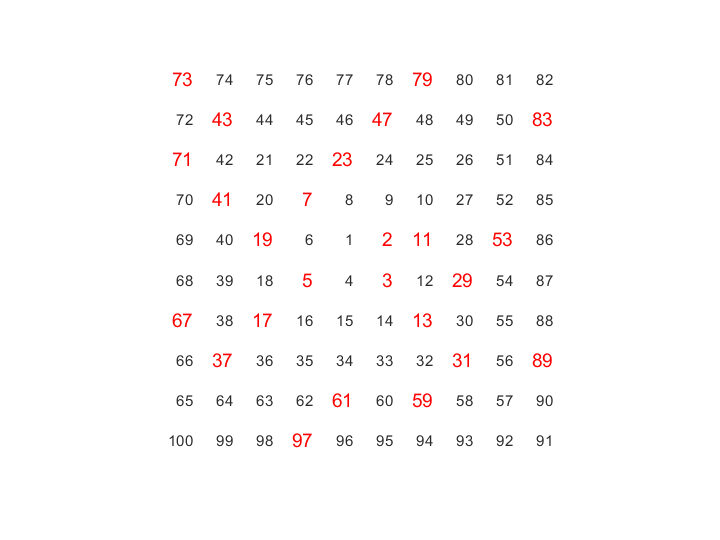Ulam's numbering at any point $(i,j)$ in the plane is a piecewise quadratic function of $i$ and $j$. For example, the values along the upper and lower halves of the antidiagonal, or their offsets by one, (depending upon whether the size is odd or even), are perfect squares. So there are certainly not any primes along these diagonals. This reminds me of the quadratics that generate primes. The most famous is Euler's polynomial, $$p(n) = n^2-n+41$$ This generates primes for $n$ from 1 through 40, but, obviously, not for $n$ equal to 41.
   n = 1:41;
p = n.^2-n+41;
isprime(p)

ans =
Columns 1 through 13
1     1     1     1     1     1     1     1     1     1     1     1     1
Columns 14 through 26
1     1     1     1     1     1     1     1     1     1     1     1     1
Columns 27 through 39
1     1     1     1     1     1     1     1     1     1     1     1     1
Columns 40 through 41
1     0

The Euler primes themselves don't show up in the Ulam prime spiral. But each of the diagonal segments corresponds to a quadratic polynomial, although probably one that generates fewer than 40 primes.

#### Improved Spiral Function

The MATLAB demos directory has a spiral function that generates Ulam's numbering. I'm afraid that the code is ancient, and pretty bad. It's not too hard to write better code. This generates successively larger spirals by rotating one of a given size 180 degrees and then appending another column at the right and another row at the bottom to produce the next larger size.
   type improved_spiral

   function S = improved_spiral(n)
% SPIRAL SPIRAL(n) is an n-by-n matrix with elements ranging
% from 1 to n^2 in a rectangular spiral pattern.
S = [];
for m = 1:n
S = rot90(S,2);
S(m,m) = 0;
p = m^2-m+1;
v = (m-1:-1:0);
S(:,m) = p-v';
S(m,:) = p+v;
end


#### Prime Spiral Is Spy

Once we have the spiral numbering, the prime spiral is our old friend, the spy plot.
   type primespiral_core.m

   function primespiral(n)
% PRIMESPIRAL PRIMESPIRAL(n) is Ulam's prime spiral.
P = zeros(n,n);
P(primes(n^2)) = 1;
S = improved_spiral(n);
P = P(S);
spy(P)


#### Increasing Sizes

Here are prime spirals of three different sizes.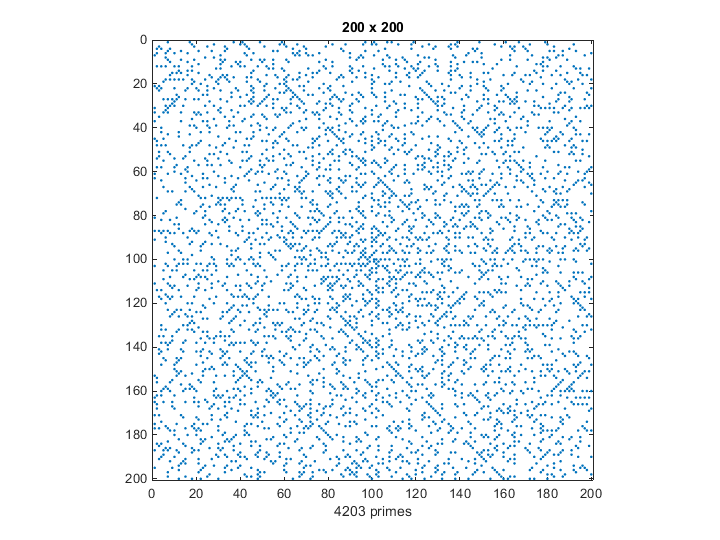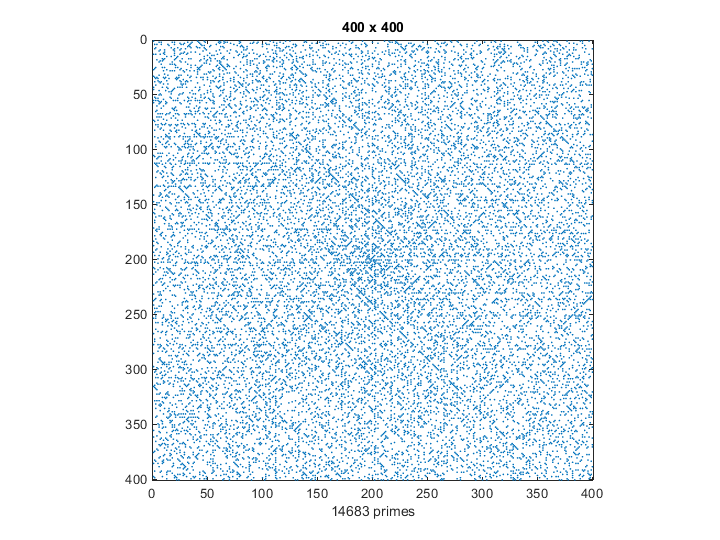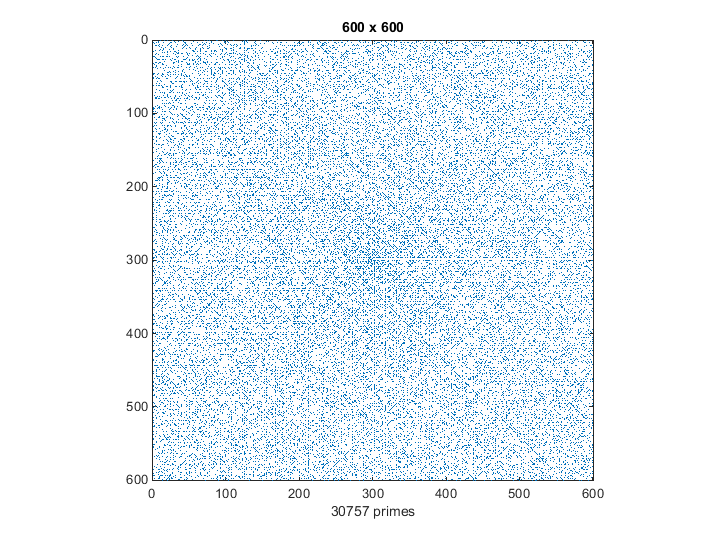#### Animated Prime Spiral

The function primespiral in my textbook Numerical Computing with MATLAB includes a provision for starting the numbering at a value larger than one. Animating this feature emphasizes the diagonal nature of the prime locations. The title on this plot is the starting value of the numbering.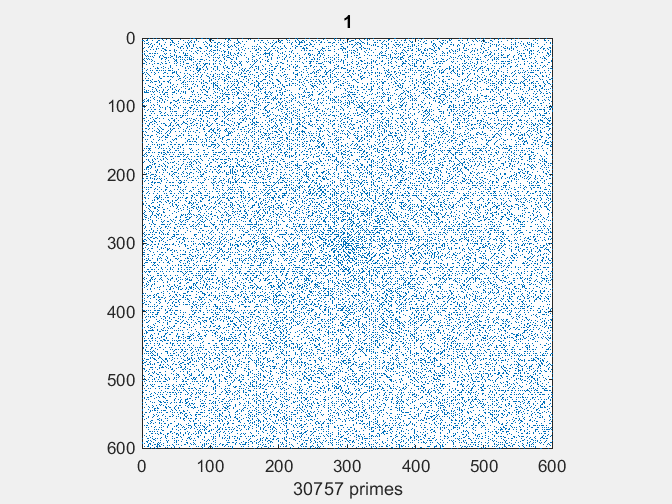Published with MATLAB® R2014b
|